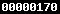GSE Algebra I GSE Geometry GSE Algebra II GSE PreCalc Other Courses Calculus I Adv. Mathematical Decision Making Analytical Geometry ```CP-Statistics ``` `All Units` Coordinate Algebra Integrated Algebra I Integrated Geometry GPS Middle School Math GIVE BACK TO MATT Home >Brain Teasers > Classics Page 5Review

Search Site:

 Page 1 Page 2 Page 3 Page 4 Page 5 Page 6

Classic Brain Teasers and Riddles

(Click on the question to see the answer.)

 61. It takes 5 builders to build 5 houses in 5 days.If the ratios remain the same, how many days should it take 100 builders to build 100 houses?
 ANSWER It should still take 5 days for 100 builders to build 100 houses if all the ratios remain the same.

 62 Three students checked into a hotel and paid the clerk \$30 for a room (\$10 each). When the hotel manager returned, he noticed that the clerk had incorrectly charged \$30 instead of \$25 for the room. The manager told the clerk to return \$5 to the students. The clerk, knowing that the students would not be able to divide \$5 evenly, decided to keep \$2 and to give them only \$3. The students were very happy because they paid only \$27 for the room (\$9 each). However, if they paid \$27 and the clerk kept \$2, that adds up to \$29. What happened to the other Dollar?
 ANSWER This is an example of adding the wrong value. The students ended up paying \$27 which came from \$30 - \$3 = \$27 and \$27 + \$3 is still a total of \$30. The \$2 the clerk kept is the additional amount the students should have also gotten back which would reduce the \$27 down to the actual price of \$25 (i.e. \$27 - \$2 = \$25), the amount the students were suppose to be charged.

63. The four points represent vertices of a square. How can you move exactly two points to create the vertices of a square of exactly twice the area?64 You're on a plane to New York City. You want to know if it's snowing and the weather app on your phone doesn't always get it right. So you call 3 of your friends who live in New York City and ask each one if it's snowing. Each friend has a 2/3 chance of telling you the truth and a 1/3 chance of lying to you for fun. All 3 friends tell you, "Yes. It is snowing here." If those probabilities are accurate, what is the probability that it is in fact snowing in New York City at the moment?
 ANSWER The chance that all 3 lied is (1/3)^3 = 1/27. So, there is only a 1/27 chance that they all lied about it snowing. So the chance that just one or more of them is telling the truth is 26/27. This is a binomial probability situation of lying versus truth. A more challenging question would be to ask what are the chances it is actually snowing if 2 friends said it was snowing and one said it wasn't.

 65 A

 66 C

 67 G

 68 T

 69 T

70.
C

71. C

 72 U

 73 U

74. W

 75 S